In :
import pymongo
import pandas as pd
import matplotlib.pyplot as plt
import seaborn as sb
import pytz
import numpy as np
import matplotlib.dates as mdates
import datetime
from IPython.display import Image
from IPython.core.display import HTML
import time

pd.set_option('precision', 2)

ist = pytz.timezone('Asia/Calcutta')
utc = pytz.timezone('UTC')

sb.set_palette("husl")

client = pymongo.MongoClient()
db = client.aircare

%matplotlib inline
# Set defaults for the pyplot styles
params = {
'legend.fontsize': 14,
'legend.handlelength': 2,
'axes.labelsize': 16,
'axes.titlesize':20,
'figure.titlesize':2
}
plt.rcParams.update(params)
ug_m3 = " μg/$\mathregular{m^3}$"
format_str = "Most polluted {0} of the {1} is {2} with PM2.5={3:.0f}μg/m3"

In :
# Which sensors to use for analysis
selected_sensor = 'rxdx'

# Sensors to benchmark against
#benchmark_sensors = None                         # Select everything
benchmark_sensors = 'plv_20'                      # Select a single benchmark sensor
#benchmark_sensors = ['plv_20', 'pm_417_2', 'gadjoy', 'imdent']       # Select a list of benchmark sensors
aqi_standards = "USA"                           # INDIA OR USA
start_date = None
end_date = None
end_date = pd.Timestamp('2018-07-30 00:00:00+05:30', tz='Asia/Calcutta')

In :
# Plot common elements for each graph
def plt_common_elements(plt, xlim=(0, None), ylim=(0,140), show_captions=True):
fig = plt.figure(figsize=(14,9))
ax = fig.gca()

# Gridlines
ax.grid(which='both', alpha=0.5, color='grey')
# Y-axis ticks
if ylim == None or ylim == None:
pass
else:
ax.set_yticks(np.arange(ylim, ylim+1, 10))

# India pollution guideline values
ug_m3_b =  ug_m3 + ')'

a = 2

if aqi_standards == "INDIA":
plt.axhline(120, color='tomato', label='India PM2.5 Poor (91-120' + ug_m3_b, linewidth=1, linestyle='dashed')
plt.axhline(90, color='darkviolet', label='India PM2.5 Moderately Polluted (61-90' + ug_m3_b, linewidth=1, linestyle='dashed')
plt.axhline(60, color='darkcyan', label='India PM2.5 Satisfactory (31-60' + ug_m3_b, linewidth=1, linestyle='dashed')
plt.axhline(30, color='g', label='India PM2.5 - Good (0-30' + ug_m3_b, linewidth=1, linestyle='dashed')
#plt.axhline(25, color='olive', label='WHO Recommendation (0-25' + + ug_m3_b, linewidth=1, linestyle='dashed')

# Show a caption in the veritical center of the guidelines
if show_captions:
plt.text(xlim, 120+a, "Very Poor", fontsize=12, color='white', backgroundcolor="r")
plt.text(xlim, 90+a, "Poor", fontsize=12,  color='white', backgroundcolor="tomato")
plt.text(xlim, 60+a, " Moderately Polluted", fontsize=12,  color='white', backgroundcolor="darkviolet")
plt.text(xlim, 30+a, " Satisfactory", fontsize=12, color='white',  backgroundcolor="darkcyan")
plt.text(xlim, 0+a, " Good", fontsize=12, color='white', backgroundcolor="g")
elif aqi_standards == "USA":
plt.axhline(150.4, color='tomato', label='US PM2.5 Unhealthy (55.5-150.4' + ug_m3_b, linewidth=2, linestyle='dashed')
plt.axhline(55.4, color='darkorange', label='US PM2.5 Unhealthy for Sensitive Groups (35.5-55.4' + ug_m3_b, linewidth=2, linestyle='dashed')
plt.axhline(35.4, color='y', label='US PM2.5 Moderate (12.1-35.4' + ug_m3_b, linewidth=2, linestyle='dashed')
plt.axhline(12, color='g', label='US PM2.5 - Good (0-12' + ug_m3_b, linewidth=2, linestyle='dashed')

if show_captions:
plt.text(xlim, 55.5+a, "Unhealthy", fontsize=12,  color='white', backgroundcolor="tomato", fontweight="bold")
plt.text(xlim, 35.5+a, "Unhealthy (S)", fontsize=12,  color='white', backgroundcolor="darkorange", fontweight="bold")
plt.text(xlim, 12.1+a, "Moderate", fontsize=12, color='white',  backgroundcolor="y", fontweight="bold")
plt.text(xlim, 0+a, "Good", fontsize=12, color='white', backgroundcolor="g", fontweight="bold")

if ylim == None or ylim == None:
pass
else:
plt.ylim(ylim, ylim)

return fig, ax

# Group data by "by" and plot the values
# We have main data in df and the bench mark data in df_bench
def plt_groups(plt=None, df=None, df_bench=None, by=None):
df_g = df.groupby(by=by).agg(agg_options)
df_bench_g = df_bench.groupby(by=by).agg(agg_options)
fig, ax = plt_common_elements(plt, ylim=(0,120))
plt.plot(df_g.index, df_g.pm25, linewidth=2, marker='o', markersize=10, label=main_label)
plt.plot(df_bench_g.index, df_bench_g.pm25, linewidth=2, marker='o', markersize=10, label=benchmark_label)
return fig, ax, df_g, df_bench_g

# The following functions are used to generate labels for the X-axis
# maplotlib could have utilitiy functions for these but...
def hours_in_a_day():
hours = []
for i in np.arange(0,24,1):
am_pm = ':00 AM'
if i >= 12:
v = i - 12
am_pm = ':00 PM'
else:
v = i

if v < 10:
if v == 0:
hours.append('12' + am_pm)
else:
hours.append('0' + str(v) + am_pm)
else:
hours.append(str(v) + am_pm)

return hours

def days_in_a_week():
return ('Mon', 'Tue', 'Wed', 'Thu', 'Fri', 'Sat', 'Sun')

# Day of week to number, where number 0 - Mon, 6 - Sun
def dow2n(name):
return days_in_a_week().index(name)

In :
# Sensor ids of different sensors
sensors = {
'rxdx':     { 'name':'RxDx', 'id':"b8:27:eb:78:c4:75"},
'pm_417_2': {'name':'APM 417/2', 'id':"b8:27:eb:cb:bf:d3"},
'plv_20':   {'name':'PLV 20', 'id':"b8:27:eb:76:b9:00"},
'imdent':   { 'name':'Imdent', 'id':"b8:27:eb:a4:78:74"}
}

benchmark_label = "PM 2.5 Benchmark"

# Aggregation options
agg_options = {'pm25':'mean', 'samples':'sum'}

# Which columns to describe
describe_columns = ['AQI', 'pm1', 'pm10', 'pm25']

analyze_sensor_id = sensors[selected_sensor]['id']
main_label = "PM 2.5 " + sensors[selected_sensor]['name']

if benchmark_sensors == None:
# Select all the sensors
benchmark_sensor_id = {'$in':[sensors[x]['id'] for x in sensors.keys() if x != selected_sensor]} elif type(benchmark_sensors) == list: # Select sensors from a list benchmark_sensor_id = {'$in':[sensors[x]['id'] for x in benchmark_sensors if  x != selected_sensor]}
else:
# Select a single sensor
benchmark_sensor_id = sensors[benchmark_sensors]['id']

In :
# Get data from Mongo DB
def get_data(sensor_id):
# Ignore the id field
project = {'_id':0 }

# Which values to project
for key in ['AQI', 'pm1', 'pm25', 'pm10', 'date']:
project[key] = '$measurement.' + key # Match only the desired sensor id pipeline = [ {'$match':{'sensor_id':sensor_id}},
{'\$project': project}
]

ret = db.command('aggregate', 'measurement', pipeline=pipeline)
df = pd.DataFrame(ret['result'])
# Create additional columns to be used later
df['date']= df.date.dt.tz_localize(utc)  # The date is in UTC
df['date'] = df.date.dt.tz_convert(ist)  # Convert to IST
df['hour'] = df.date.dt.hour             # 0 to 23
df['minute'] = df.date.dt.minute         # 0 to 59
df['dayofyear'] = df.date.dt.dayofyear   # 1 to 365
df['dow'] = df.date.dt.dayofweek         # 0 (Mon) to 6 (Sun)
df['samples'] = 1                        # Number of samples to sum up for grouping ops
return df
!date

Sun Aug 12 15:38:09 IST 2018AirCare by MapsHalli

# RxDx Air Quality Analysis - July 30-2018¶

## 1. Executive Summary¶

Air quality data was collected from AirCare sensor during the period July-20-2018 to July-30-2018.

Location near RxDx has 3.5x times the pollution of other locations in Whitefield. The average PM2.5 value for the measurement period was 42μg/m3.

During the data collection period, the 24 hours PM2.5 average for the worst day at RxDx was 68μg/m3. At the same time it was 9μg/m3 from another AirCare sensor (benchmark sensor) at Ramagondanahalli. The WHO recommended annual average value for PM2.5 is 25μg/m3. There is no safe level for PM2.5. A long term exposure of PM2.5 at 40μg/m3 is associated with an increase in the long-term risk of cardiopulmonary mortality by 24-52% and lung cancer mortality by 32%.

The PM2.5 values at RxDx shows a huge variation of 19μg/m3 when compared to 4μg/m3 at the benchmark location.

Based on the pattern of pollution readings and visual observations, the pollution is caused by one or more nearby industries.

## 2. Descriptive stats¶

### RxDx location¶

In :
df = get_data(sensor_id=analyze_sensor_id)
df_bench = get_data(sensor_id=benchmark_sensor_id)
if start_date == None:
if df.date.min() > df_bench.date.min():
start_date = df.date.min()
else:
start_date = df_bench.date.min()

if end_date == None:
if df.date.max() < df_bench.date.max():
end_date = df.date.max()
else:
end_date = df_bench.date.max()

print("Analyis period, from:", start_date, "to:", end_date)
df = df[(df.date >= start_date) & (df.date <= end_date)].copy()
df_bench = df_bench[(df_bench.date >= start_date) & (df_bench.date <= end_date)].copy()

df[describe_columns].describe()

Analyis period, from: 2018-07-20 14:10:37.335000+05:30 to: 2018-07-30 00:00:00+05:30

Out:
AQI pm1 pm10 pm25
count 2699.00 2699.00 2699.00 2699.00
mean 112.56 27.13 55.70 42.89
std 29.63 14.05 24.68 20.93
min 46.00 6.00 13.00 11.00
25% 91.00 19.00 41.00 31.00
50% 107.00 24.00 50.00 38.00
75% 129.00 30.00 64.00 47.00
max 305.00 185.00 272.00 255.00

### Benchmark location¶

In :
df_bench[describe_columns].describe()

Out:
AQI pm1 pm10 pm25
count 2701.00 2701.00 2701.00 2701.00
mean 53.11 9.01 18.58 14.08
std 12.57 3.28 5.56 4.55
min 8.00 1.00 5.00 2.00
25% 46.00 7.00 15.00 11.00
50% 55.00 9.00 18.00 14.00
75% 61.00 11.00 22.00 17.00
max 137.00 30.00 65.00 50.00

## 3. Data Distribution¶

In :
plt_common_elements(plt, xlim=(0.55,None), ylim=(None, None))
plt.boxplot([df.pm25, df_bench.pm25], labels=[sensors[selected_sensor]['name'], 'Benchmark'], whis='range' )
plt.ylabel('PM 2.5' + ug_m3)
plt.legend(loc='upper right')
plt.show()

plt_common_elements(plt, ylim=(0,160))
plt.hist([df.pm25], orientation='horizontal', color='burlywood', histtype='stepfilled', alpha=0.95)
plt.ylabel('PM 2.5' + ug_m3)
plt.xlabel('Number of samples')
plt.legend(loc='upper right')
pass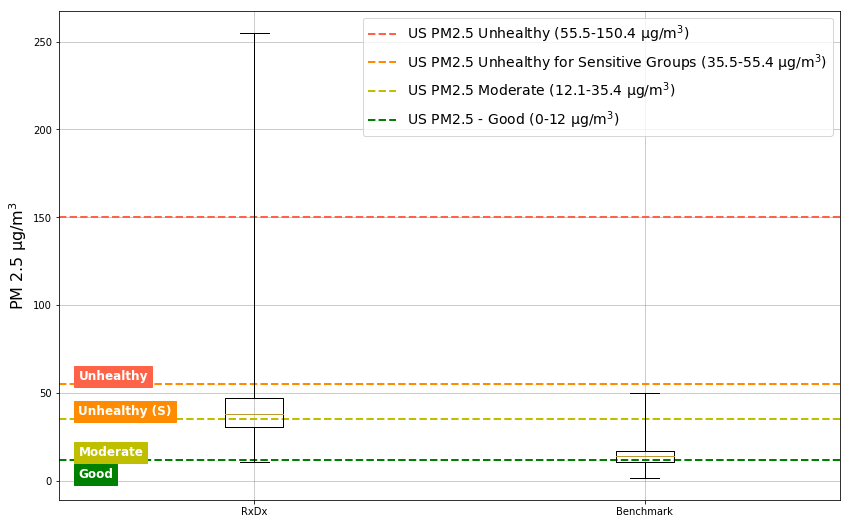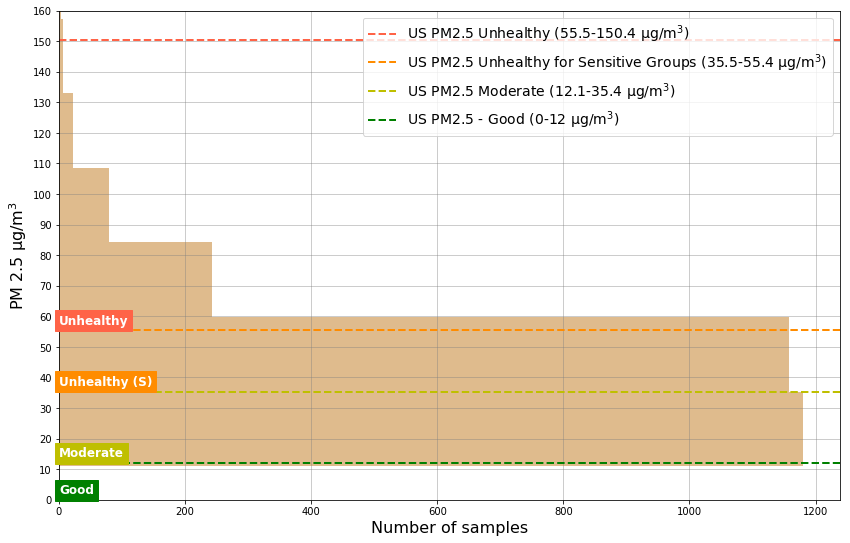## 4. Samples¶

The plotted samples show the PM2.5 values. Notice the high amount of variation exhibited by the RxDx sensor. This indicates periodic pollution activity nearby causing such "puffing" pollution.

In :
idxmax = df.pm25.idxmax()
for opt in [(None, None, 1, '.'), (idxmax - 12*3, idxmax + 12 * 3, 3, 'o')]:
plt_common_elements(plt, ylim=(None,None))
if opt == None:
if len(df) < len(df_bench):
count = len(df)
else:
count = len(df_bench)
begin = 0
end = count
else:
begin = opt
end = opt

plt.plot(range(0, end - begin), df[begin:end].pm25, linewidth=opt, label=main_label, marker=opt )
plt.plot(range(0, end - begin), df_bench[begin:end].pm25, linewidth=opt, label=benchmark_label, marker=opt)

plt.ylabel('PM 2.5' + ug_m3)

if opt == None:
plt.xlabel('Measurement Sequence')
plt.legend(loc='center')
plt.title('PM2.5 values for all ' + str(count) + ' measurements')
else:
plt.title('PM2.5 values surrounding peak PM2.5 incident')
plt.xlabel('72 measurements (6 hours)')
plt.show()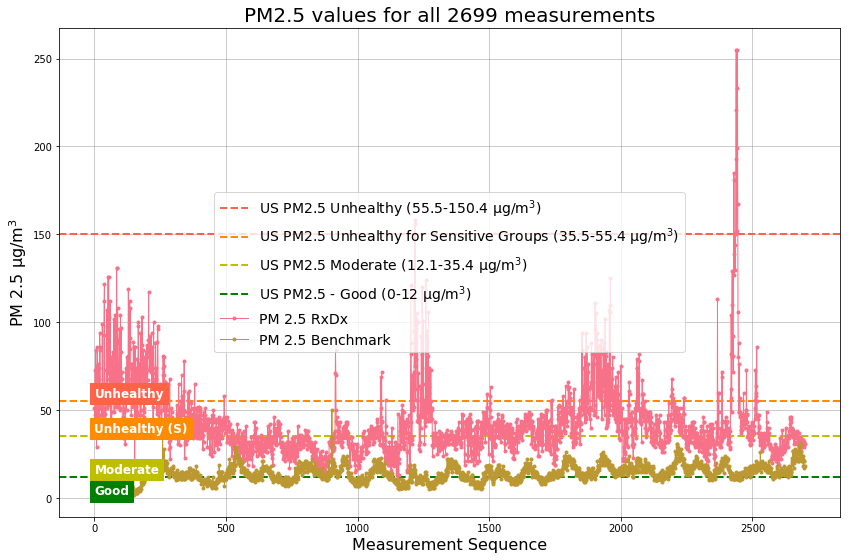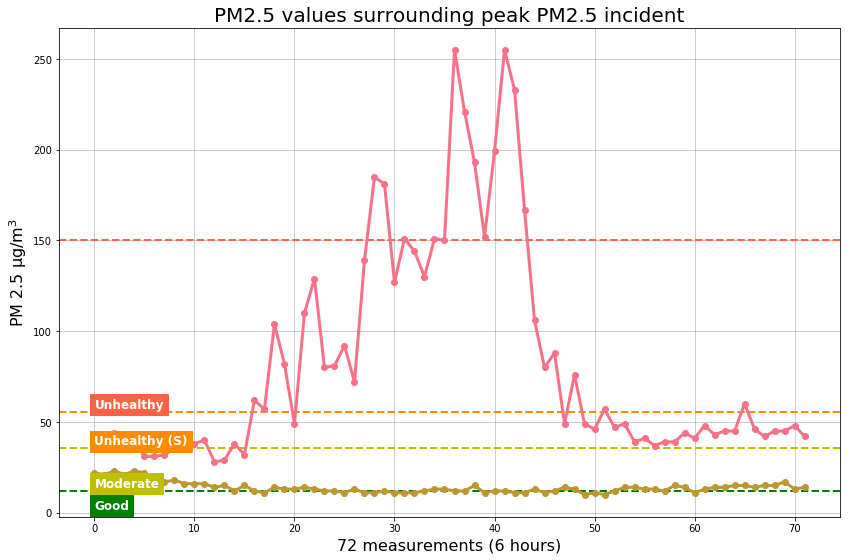## 5. Day pollution¶

In :
def doy_to_date(v):
return pd.Timestamp('01/01/2018') + pd.Timedelta(v-1, unit='D')

df_day_of_year = df.groupby(by='dayofyear').agg(agg_options)
df_day_of_year['doy'] = df_day_of_year.index
df_day_of_year['mdate'] = df_day_of_year['doy'].apply(doy_to_date)

df_bench_day_of_year = df_bench.groupby(by='dayofyear').agg(agg_options)
df_bench_day_of_year['doy'] = df_bench_day_of_year.index
df_bench_day_of_year['mdate'] = df_bench_day_of_year['doy'].apply(doy_to_date)

# We want to show benchmark data only those days for which data is available
clip = -len(df_day_of_year)
clip = 0

# Set x-axis min and max to one day before the first date and one day after the last date respectively
xlim = ( df_bench_day_of_year['mdate'].iloc[clip]  -  pd.Timedelta(1, unit='D'),
df_bench_day_of_year['mdate'].iloc[-1] + pd.Timedelta(1, unit='D'))

fig, ax = plt_common_elements(plt, xlim=xlim, ylim=(None, None))
plt.plot(df_day_of_year.mdate, df_day_of_year.pm25, linewidth=2, marker='o', markersize=10, label=main_label)
plt.plot(df_bench_day_of_year[clip:].mdate, df_bench_day_of_year[clip:].pm25, linewidth=2, marker='o',
markersize=10, label=benchmark_label)

ax.xaxis.set_major_formatter(mdates.DateFormatter('%a %d-%B'))
ax.xaxis.set_major_locator(mdates.DayLocator())
ax.set_xlim(xlim)

plt.xlabel('Date')
plt.ylabel('Average PM 2.5' + ug_m3)
plt.title('Average PM2.5 Vs Date')
plt.gcf().autofmt_xdate()
plt.legend(loc='upper right')
most_polluted_day = doy_to_date(df_day_of_year['pm25'].idxmax()).strftime('%a %d-%B-%y')
print(format_str.format('day', 'measurement period',
most_polluted_day,
df_day_of_year['pm25'].max()))

plt.show()
pass

Most polluted day of the measurement period is Fri 20-July-18 with PM2.5=68μg/m3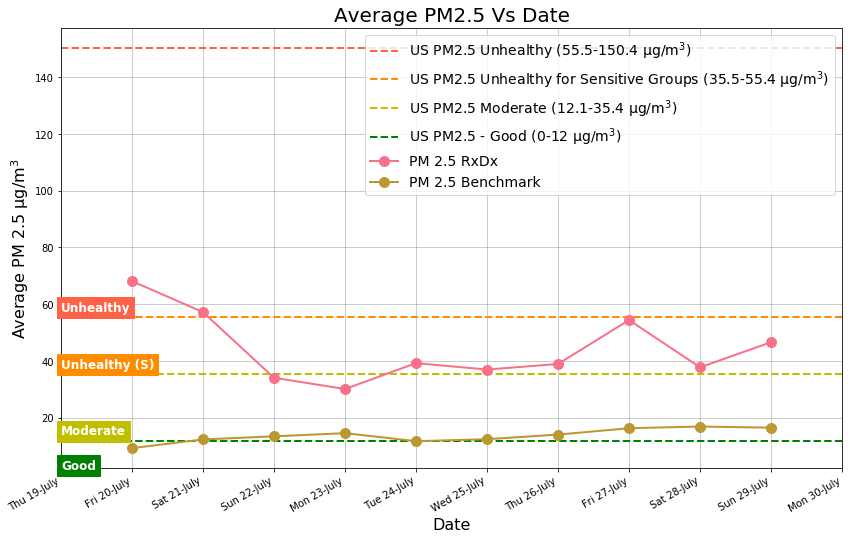## 6. Day of the week pollution¶

In :
fig, ax, df_g, df_bench_g  = plt_groups(plt=plt, df=df, df_bench=df_bench, by='dow')
plt.xlabel('Day of the Week')
plt.ylabel('Average PM 2.5' + ug_m3)
plt.xticks(np.arange(0,8,1), days_in_a_week())
plt.title('PM2.5 vs Day of the Week')
plt.legend()
most_polluted_dow = df_g['pm25'].idxmax()
print(format_str.format('day', 'week', days_in_a_week()[most_polluted_dow], df_g['pm25'].max()))
pass

Most polluted day of the week is Fri with PM2.5=58μg/m3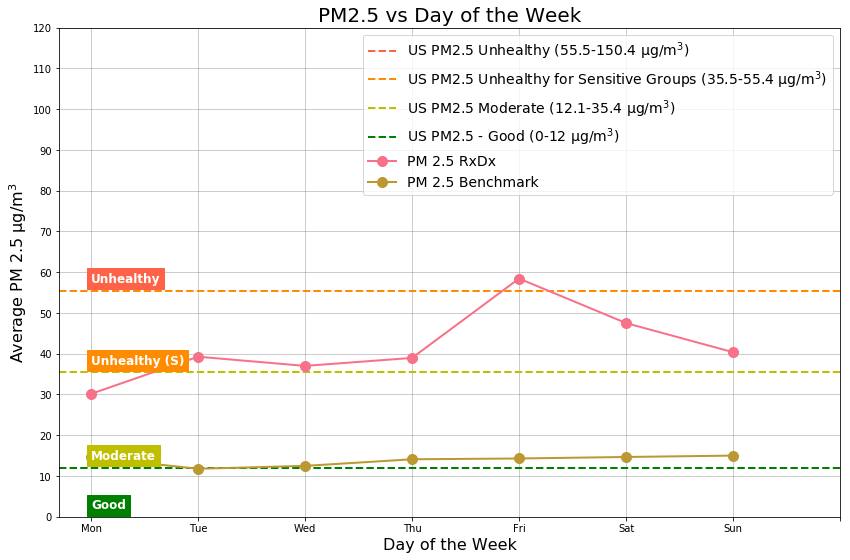## 7. Hour of the day pollution for the most polluted day of the week¶

In :
fig, ax, df_g, df_bench_g = plt_groups(plt=plt, # df=df, df_bench=df_bench, by='hour')
df=df[df.dow == most_polluted_dow],
df_bench=df_bench[df_bench.dow == most_polluted_dow],
by='hour')
plt.xlabel('Hour of the Day')
plt.ylabel('Average PM 2.5' + ug_m3)
plt.xticks(np.arange(0, 24, 1), hours_in_a_day())
plt.xticks(rotation=40)
plt.title('Average PM2.5 Vs Hour of the Day')
plt.legend()
print(format_str.format('day', 'week', days_in_a_week()[most_polluted_dow], df_g['pm25'].max()))
print(format_str.format('hour', 'day', hours_in_a_day()[df_g['pm25'].idxmax()], df_g['pm25'].max()))
pass

Most polluted day of the week is Fri with PM2.5=77μg/m3
Most polluted hour of the day is 05:00 AM with PM2.5=77μg/m3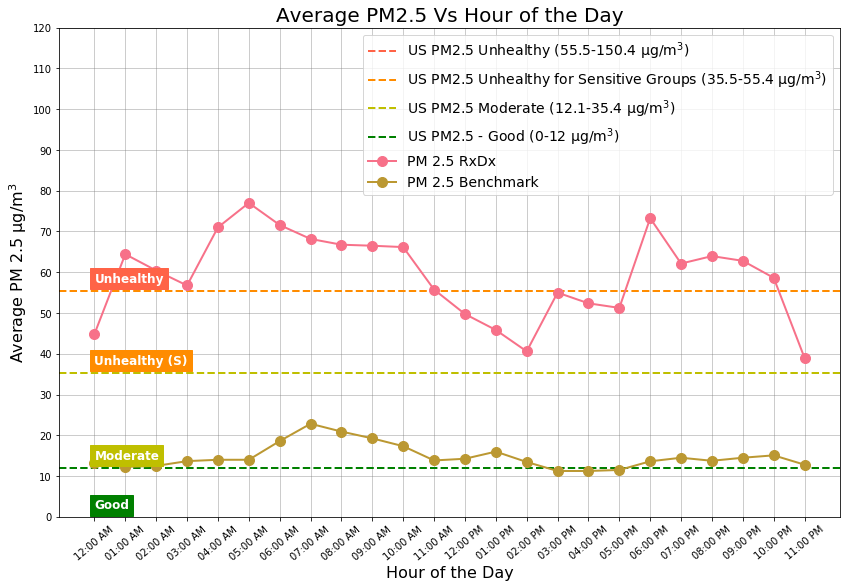## 8. Minute of the hour pollution for the most polluted day of the week¶

In :
fig, ax, df_g, df_bench_g = plt_groups(plt=plt,
df=df[df.dow == most_polluted_dow],
df_bench=df_bench[df_bench.dow == most_polluted_dow],
by='minute')
plt.xlabel('Minute of the Hour')
plt.ylabel('Average PM 2.5' + ug_m3)
plt.xticks(np.arange(0, 60, 5))
plt.title('PM2.5 vs Minute of the Hour')
plt.legend()
print(format_str.format('day', 'week', days_in_a_week()[most_polluted_dow], df_g['pm25'].max()))
print(format_str.format('minute', 'hour', df_g['pm25'].idxmax(), df_g['pm25'].max()))
pass

Most polluted day of the week is Fri with PM2.5=63μg/m3
Most polluted minute of the hour is 10 with PM2.5=63μg/m3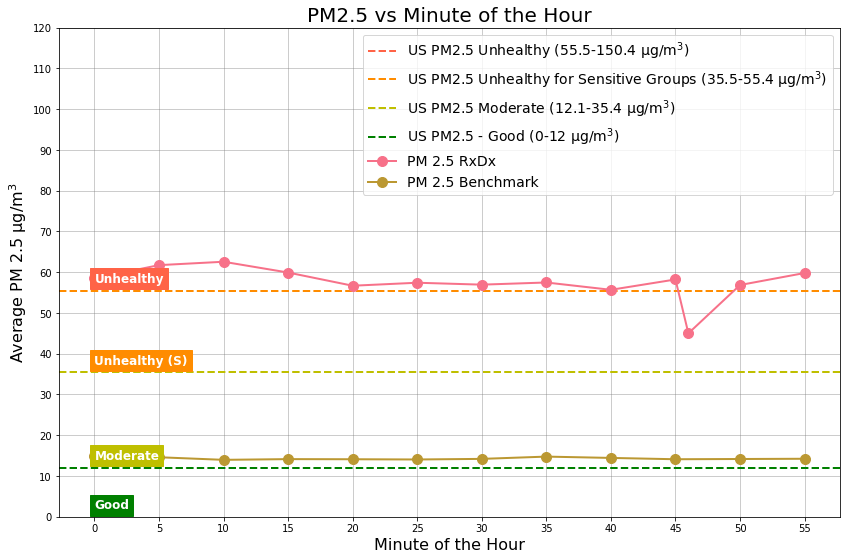## 9. Conclusion¶

Location near RxDx is polluted 3.5x times when compared to other locations in Whitefield. This location is the most polluted in Whitefield based on the data from total of 5 sensors. The pollution should be addressed at the source by installting appropriate air filters and by changing the quantity and timeline of pollution causing operations.

## 10. Disclaimer¶

This data and analysis is prepared based on the data collected from PMS3003 low cost air quality sensor. This sensor has been calibrated in the factory and has not been calibrated for humidity and temperature. If you have any questions, please contact shiv@mapshalli.org (+91-9845209952).

http://blog.mapshalli.org/index.php/aircare/